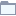# Prediction

This category contains 18 nodes.

##### Spark Decision Tree Learner

Trains a Decision Tree classification model in Spark.

##### Spark Decision Tree Learner (MLlib)

Decision tree induction performed in Spark.

##### Spark Decision Tree Learner (Regression)

Trains a regression model in Spark.

##### Spark Gradient Boosted Trees Learner

Trains a Gradient Boosted Trees classification model in Spark.

##### Spark Gradient Boosted Trees Learner (Regression)

Trains a Gradient Boosted Trees regression model in Spark.

##### Spark Gradient-Boosted Trees Learner (MLlib)

Gradient-Boosted Trees (GBTs) induction performed in Spark.

##### Spark Linear Regression Learner

Trains a linear regression model in Spark.

##### Spark Linear Regression Learner (MLlib)

Linear regression model learning performed in Spark.

##### Spark Linear SVM Learner (MLlib)

Linear SVM model learning performed in Spark.

##### Spark Logistic Regression Learner

Trains a logistic regression model in Spark.